It is to be noted that division of service channels happen in regards to number of servers that are present at each of the queues that are formed.

Poisson distribution determines the number of arrivals on a per unit time basis, where mean arrival rate is denoted by λ. As the simplest model that is used for this system, one has to check out certain assumptions that are made to ensure that this model provides correct results.

1. It is assumed that cases of reneging do not occur and everyone patiently waits in queue to get served.
2. The rate of arrival is consistent and arrival of every new candidate is taken independently.
3. The usual First Come First Serve format is followed.
4. Though service rate is not known but arrival rate follows Poisson distribution format.
5. As the queue keeps receding over a certain time period, it can be taken as average service rate is greater than average arrival rate.
6. Negative exponential probability distribution helps in depicting service time.

It is based on these assumptions that further mathematical problems are based, solving which would give students a better insight into details associated with this theory.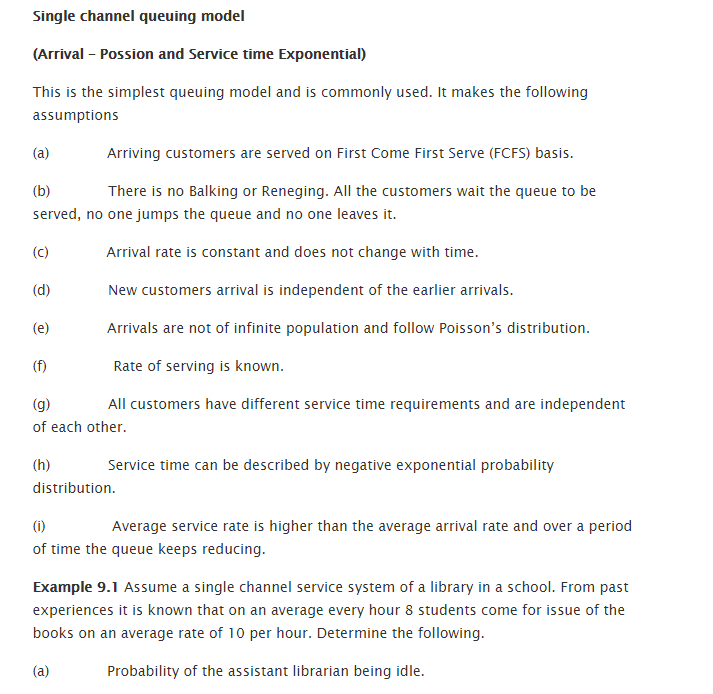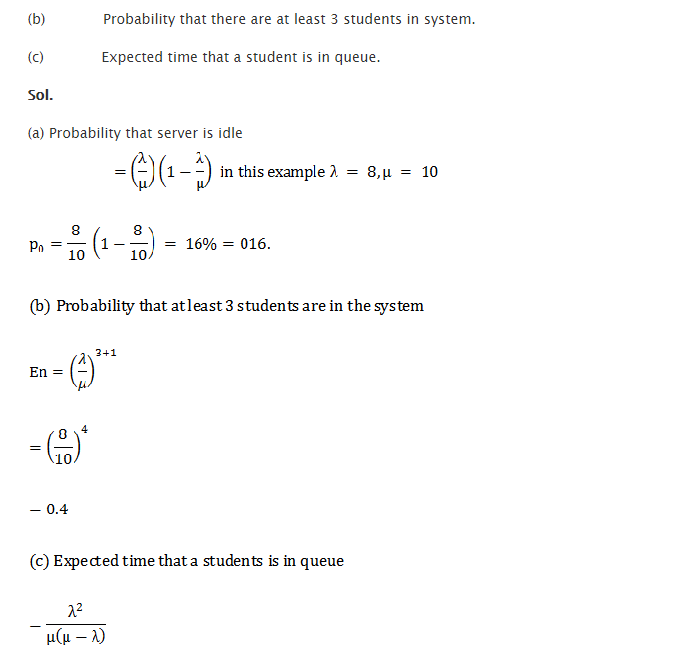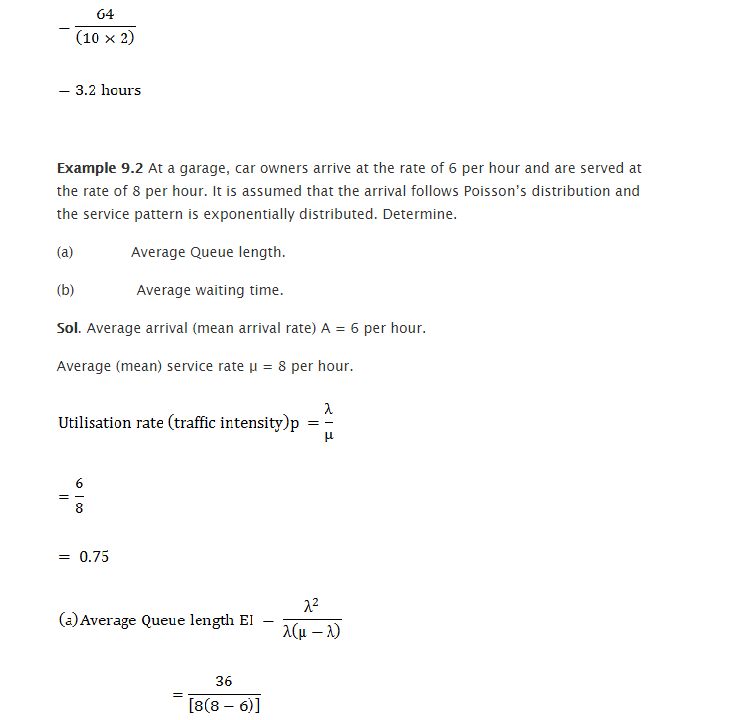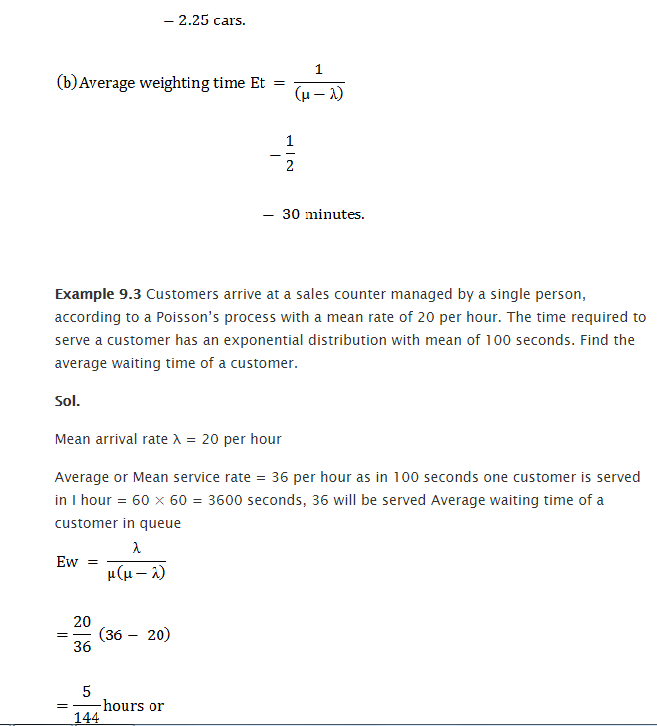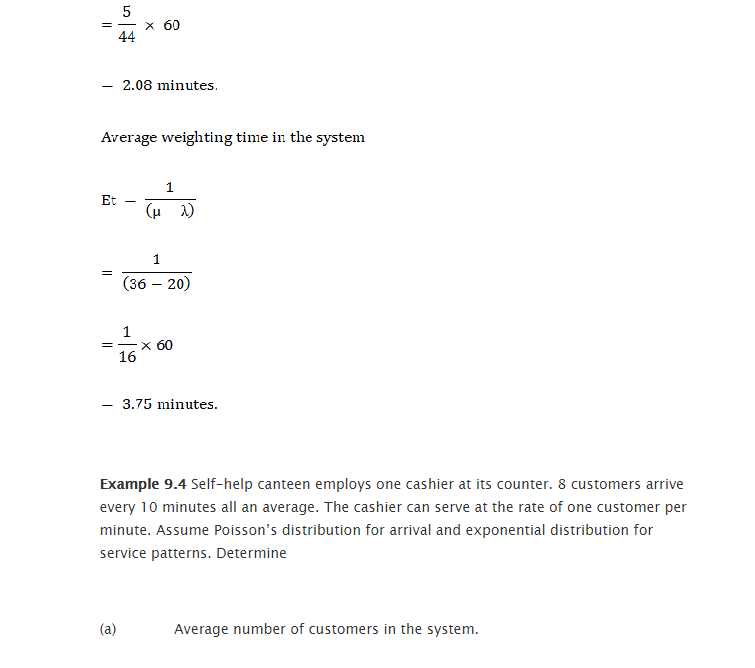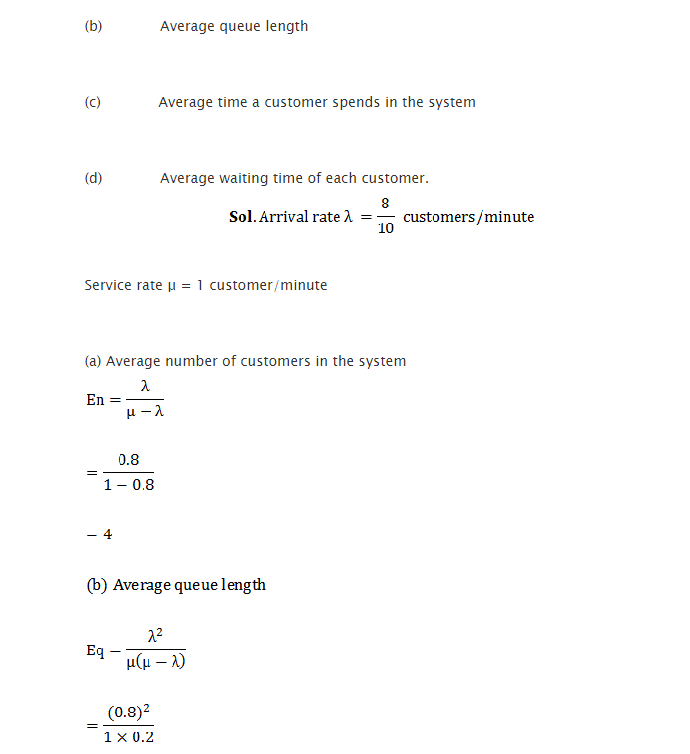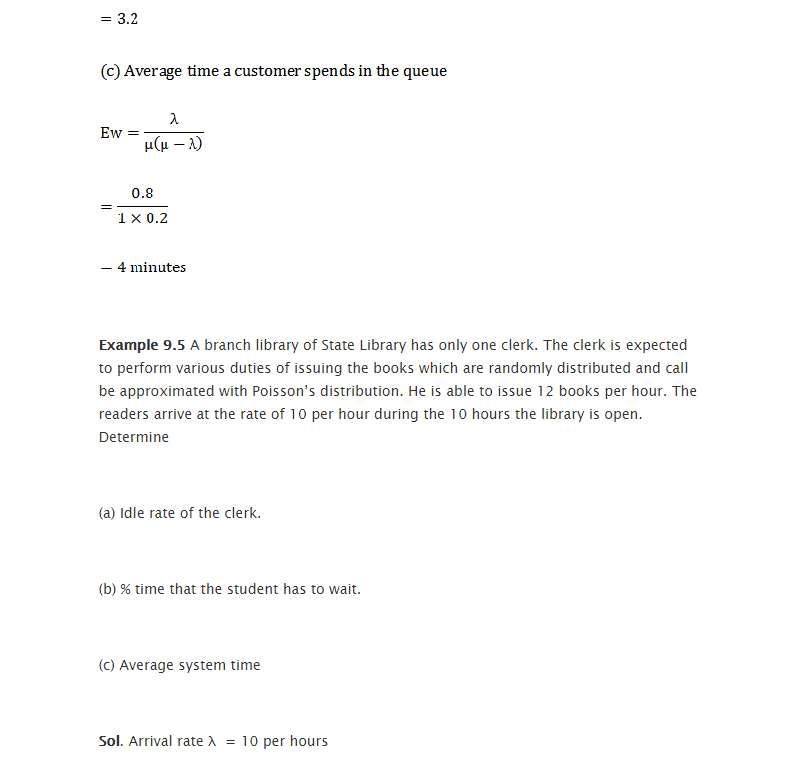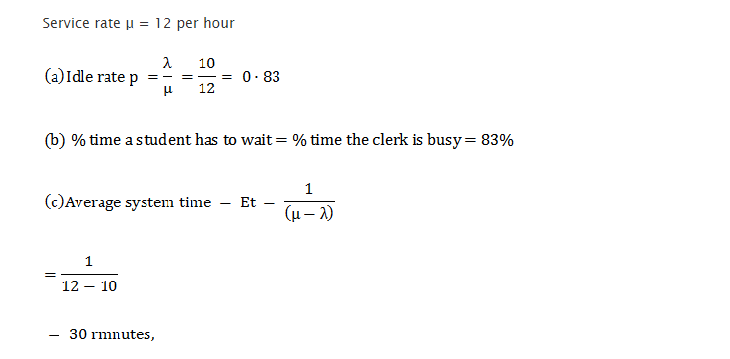### Customer Reviews

My Homework Help
Rated 5.0 out of 5 based on 510 customer reviews at